## Twitter Text Mining with Python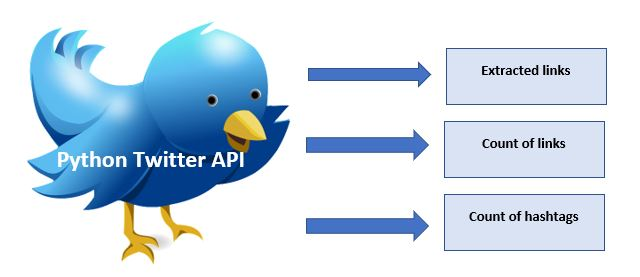In this post (and few following posts) we will look how to get interesting information by extracting links from results of Twitter search by keywords and using machine learning text mining. While there many other posts on the same topic, we will cover also additional small steps that are needed to process data. This includes such tasks as unshorting urls, setting date interval, saving or reading information.

Below we will focus on extracting links from results of Twitter search API python.

## Getting Login Information for Twitter API

The first step is set up application on Twitter and get login information. This is already described in some posts on the web .
Below is the code snippet for this:

`your code here`
```import tweepy as tw

CONSUMER_KEY ="xxxxx"
CONSUMER_SECRET ="xxxxxxx"
OAUTH_TOKEN = "xxxxx"
OAUTH_TOKEN_SECRET = "xxxxxx"

auth = tw.OAuthHandler(CONSUMER_KEY, CONSUMER_SECRET)
auth.set_access_token(OAUTH_TOKEN, OAUTH_TOKEN_SECRET)
api = tw.API(auth, wait_on_rate_limit=True)
```

## Defining the Search Values

Now you can do search by keywords or hashtags and get tweets.
When we do search we might want to specify the start day so it will give results dated on this start day or after.

For this we can code as the following:

```from datetime import datetime
from datetime import timedelta

NUMBER_of_TWEETS = 20
SEARCH_BEHIND_DAYS=60
today_date=datetime.today().strftime('%Y-%m-%d')

today_date_datef = datetime.strptime(today_date, '%Y-%m-%d')
start_date = today_date_datef - timedelta(days=SEARCH_BEHIND_DAYS)

for search_term in search_terms:
tweets = tw.Cursor(api.search,
q=search_term,
lang="en",
since=SEARCH_BEHIND_DAYS).items(NUMBER_of_TWEETS)
```

The above search will return 20 tweets and will look only within 60 days from day of search. If we want use fixed date we can replace with since=’2019-12-01′

## Processing Extracted Links

Once we got tweets text we can extract links. However we will get different types of links. Some are internal twitter links, some are shorten, some are regular urls.

So here is the function to sort out the links. We do not need internal links – the links that belong to Twitter navigation or other functionality.

```try:
import urllib.request as urllib2
except ImportError:
import urllib2

import http.client
import urllib.parse as urlparse

def unshortenurl(url):
parsed = urlparse.urlparse(url)
h = http.client.HTTPConnection(parsed.netloc)
h.request('HEAD', parsed.path)
response = h.getresponse()
if response.status >= 300 and response.status < 400 and response.getheader('Location'):
return response.getheader('Location')
else: return url
```

Once we got links we can save urls information in csv file. Together with the link we save twitter text, date.
Additionally we count number of hashtags and links and also save this information into csv files. So the output of program is 3 csv files.

## Conclusion

Looking in the output file we can quickly identify the links of interest. For example just during the testing this script I found two interesting links that I was not aware. In the following post we will look how to do even more automation for finding cool links using Twitter text mining.

Below you can find full source code and the references to web resources that were used for this post or related to this topic.

```# -*- coding: utf-8 -*-

import tweepy as tw
import re
import csv

from datetime import datetime
from datetime import timedelta

NUMBER_of_TWEETS = 20
SEARCH_BEHIND_DAYS=60
today_date=datetime.today().strftime('%Y-%m-%d')

today_date_datef = datetime.strptime(today_date, '%Y-%m-%d')
start_date = today_date_datef - timedelta(days=SEARCH_BEHIND_DAYS)
try:
import urllib.request as urllib2
except ImportError:
import urllib2

import http.client
import urllib.parse as urlparse

def unshortenurl(url):
parsed = urlparse.urlparse(url)
h = http.client.HTTPConnection(parsed.netloc)
h.request('HEAD', parsed.path)
response = h.getresponse()
if response.status >= 300 and response.status < 400 and response.getheader('Location'):
return response.getheader('Location')
else: return url

CONSUMER_KEY ="xxxxx"
CONSUMER_SECRET ="xxxxxxx"
OAUTH_TOKEN = "xxxxxxxx"
OAUTH_TOKEN_SECRET = "xxxxxxx"

auth = tw.OAuthHandler(CONSUMER_KEY, CONSUMER_SECRET)
auth.set_access_token(OAUTH_TOKEN, OAUTH_TOKEN_SECRET)
api = tw.API(auth, wait_on_rate_limit=True)
# Create a custom search term

search_terms=["#chatbot -filter:retweets",
"#chatbot+machine_learning -filter:retweets",
"#chatbot+python -filter:retweets",
"text classification -filter:retweets",
"text classification python -filter:retweets",
"machine learning applications -filter:retweets",
"sentiment analysis python  -filter:retweets",
"sentiment analysis  -filter:retweets"]

def count_urls():
url_counted = dict()
url_count = dict()
with open('data.csv', 'r', encoding="utf8" ) as csvfile:
line = csvfile.readline()
while line != '':  # The EOF char is an empty string

line = csvfile.readline()
items=line.split(",")
if len(items) < 3 :
continue

url=items
twt=items
# key =  Tweet and Url
key=twt[:30] + "___" + url

if key not in url_counted:
url_counted[key]=1
if url in url_count:
url_count[url] += 1
else:
url_count[url] = 1
print_count_urls(url_count)

def print_count_urls(url_count_data):

for key, value in url_count_data.items():
print (key, "=>", value)

with open('data_url_count.csv', 'w', encoding="utf8", newline='' ) as csvfile_link_count:
fieldnames = ['URL', 'Count']
writer = csv.DictWriter(csvfile_link_count, fieldnames=fieldnames)
writer.writeheader()

for key, value in url_count_data.items():
writer.writerow({'URL': key, 'Count': value })

def extract_hash_tags(s):
return set(part[1:] for part in s.split() if part.startswith('#'))

def save_tweet_info(tw, twt_dict, htags_dict ):

if tw not in twt_dict:
htags=extract_hash_tags(tw)
twt_dict[tw]=1
for ht in htags:
if ht in htags_dict:
htags_dict[ht]=htags_dict[ht]+1
else:
htags_dict[ht]=1

def print_count_hashtags(htags_count_data):

for key, value in htags_count_data.items():
print (key, "=>", value)

with open('data_htags_count.csv', 'w', encoding="utf8", newline='' ) as csvfile_link_count:
fieldnames = ['Hashtag', 'Count']
writer = csv.DictWriter(csvfile_link_count, fieldnames=fieldnames)
writer.writeheader()

for key, value in htags_count_data.items():
writer.writerow({'Hashtag': key, 'Count': value })

tweet_dict = dict()
hashtags_dict = dict()

for search_term in search_terms:
tweets = tw.Cursor(api.search,
q=search_term,
lang="en",
#since='2019-12-01').items(40)
since=SEARCH_BEHIND_DAYS).items(NUMBER_of_TWEETS)

with open('data.csv', 'a', encoding="utf8", newline='' ) as csvfile:
fieldnames = ['Search', 'URL', 'Tweet', 'Entered on']
writer = csv.DictWriter(csvfile, fieldnames=fieldnames)
writer.writeheader()

for tweet in tweets:
urls = re.findall('http[s]?://(?:[a-zA-Z]|[0-9]|[\$-_@.&+]|[!*\(\),]|(?:%[0-9a-fA-F][0-9a-fA-F]))+', tweet.text)

save_tweet_info(tweet.text, tweet_dict, hashtags_dict )
for url in urls:
try:
res = urllib2.urlopen(url)
actual_url = res.geturl()

if ( ("https://twitter.com" in actual_url) == False):

if len(actual_url) < 32:
actual_url =unshortenurl(actual_url)
print (actual_url)

writer.writerow({'Search': search_term, 'URL': actual_url, 'Tweet': tweet.text, 'Entered on': today_date })

except:
print (url)

print_count_hashtags(hashtags_dict)
count_urls()
```

References

## Document Similarity in Machine Learning Text Analysis with ELMo

In this post we will look at using ELMo for computing similarity between text documents. Elmo is one of the word embeddings techniques that are widely used now. In the previous post we used TF-IDF for calculating text documents similarity. TF-IDF is based on word frequency counting. Both techniques can be used for converting text to numbers in information retrieval machine learning algorithms.The good tutorial that explains how ElMo is working and how it is built is Deep Contextualized Word Representations with ELMo
Another resource is at ELMo

We will however focus on the practical side of computing similarity between text documents with ELMo. Below is the code to accomplish this task. To compute elmo embeddings I used function from Analytics Vidhya machine learning post at learn-to-use-elmo-to-extract-features-from-text/

We will use cosine_similarity module from sklearn to calculate similarity between numeric vectors. It computes cosine similarity between samples in X and Y as the normalized dot product of X and Y.

```# -*- coding: utf-8 -*-

from sklearn.metrics.pairwise import cosine_similarity

import tensorflow_hub as hub
import tensorflow as tf

elmo = hub.Module("https://tfhub.dev/google/elmo/2", trainable=True)

def elmo_vectors(x):

embeddings=elmo(x, signature="default", as_dict=True)["elmo"]

with tf.Session() as sess:
sess.run(tf.global_variables_initializer())
sess.run(tf.tables_initializer())
# return average of ELMo features
return sess.run(tf.reduce_mean(embeddings,1))
```

Our data input will be the same as in previous post for TF-IDF: collection the sentences as an array. So each document here is represented just by one sentence.

```corpus=["I'd like an apple juice",
"An apple a day keeps the doctor away",
"Eat apple every day",
"We buy apples every week",
"We use machine learning for text classification",
"Text classification is subfield of machine learning"]

```

Below we do elmo embedding for each document and create matrix for all collection. If we print elmo_embeddings for i=0 we will get word embeddings vector [ 0.02739557 -0.1004054 0.12195794 … -0.06023929 0.19663551 0.3809018 ] which is numeric representation of the first document.

```elmo_embeddings=[]
print (len(corpus))
for i in range(len(corpus)):
print (corpus[i])
elmo_embeddings.append(elmo_vectors([corpus[i]]))

```

Finally we can print embeddings and similarity matrix

```print ( elmo_embeddings)
print(cosine_similarity(elmo_embeddings, elmo_embeddings))

[array([ 0.02739557, -0.1004054 ,  0.12195794, ..., -0.06023929,
0.19663551,  0.3809018 ], dtype=float32), array([ 0.08833811, -0.21392687, -0.0938901 , ..., -0.04924499,
0.08270906,  0.25595033], dtype=float32), array([ 0.45237526, -0.00928468,  0.5245862 , ...,  0.00988374,
-0.03330074,  0.25460464], dtype=float32), array([-0.14745474, -0.25623208,  0.20231596, ..., -0.11443609,
-0.03759   ,  0.18829307], dtype=float32), array([-0.44559947, -0.1429281 , -0.32497618, ...,  0.01917108,
-0.29726124, -0.02022664], dtype=float32), array([-0.2502797 ,  0.09800234, -0.1026585 , ..., -0.22239089,
0.2981896 ,  0.00978719], dtype=float32)]

The similarity matrix computed as :
[[0.9999998  0.609864   0.574287   0.53863835 0.39638174 0.35737067]
[0.609864   0.99999976 0.6036072  0.5824003  0.39648792 0.39825168]
[0.574287   0.6036072  0.9999998  0.7760986  0.3858403  0.33461633]
[0.53863835 0.5824003  0.7760986  0.9999995  0.4922789  0.35490626]
[0.39638174 0.39648792 0.3858403  0.4922789  0.99999976 0.73076516]
[0.35737067 0.39825168 0.33461633 0.35490626 0.73076516 1.0000002 ]]
```

Now we can compare this similarity matrix with matrix obtained with TF-IDF in prev post. Obviously they are different.

Thus, we calculated similarity between textual documents using ELMo. This post and previous post about using TF-IDF for the same task are great machine learning exercises. Because we use text conversion to numbers, document similarity in many algorithms of information retrieval, data science or machine learning.

## Document Similarity in Machine Learning Text Analysis with TF-IDF

Despite of the appearance of new word embedding techniques for converting textual data into numbers, TF-IDF still often can be found in many articles or blog posts for information retrieval, user modeling, text classification algorithms, text analytics (extracting top terms for example) and other text mining techniques.In this text we will look what is TF-IDF, how we can calculate TF-IDF, retrieve calculated values in different formats and how we compute similarity between 2 text documents using TF-IDF technique.

tf–idf is term frequency–inverse document frequency, is a numerical statistic that is intended to reflect how important a word is to a document in a collection or corpus. The tf–idf value increases proportionally to the number of times a word appears in the document and is offset by the number of documents in the corpus that contain the word, which helps to adjust for the fact that some words appear more frequently in general.

Here we will look how we can convert text corpus of documents to numbers and how we can use above technique for computing document similarity.

We will use sklearn.feature_extraction.text.TfidfVectorizer from python scikit-learn library for calculating tf-idf. TfidfVectorizer converts a collection of raw documents to a matrix of TF-IDF features.

We need to provide text documents as input, all other input parameters are optional and have default values or set to None. 

Here is the list of inputs from documentation:

input=’content’, encoding=’utf-8’, decode_error=’strict’, strip_accents=None, lowercase=True, preprocessor=None,
tokenizer=None, analyzer=’word’, stop_words=None, token_pattern=’(?u)\b\w\w+\b’, ngram_range=(1, 1),
max_df=1.0, min_df=1, max_features=None, vocabulary=None, binary=False,dtype=, norm=’l2’,
use_idf=True, smooth_idf=True, sublinear_tf=False)

Our text documents will be represented just one sentence and all documents will be inputted as via array corpus.
Below code demonstrates how to get document similarity matrix.

```# -*- coding: utf-8 -*-

from sklearn.feature_extraction.text import TfidfVectorizer

from sklearn.metrics.pairwise import cosine_similarity
import pandas as pd

corpus=["I'd like an apple juice",
"An apple a day keeps the doctor away",
"Eat apple every day",
"We buy apples every week",
"We use machine learning for text classification",
"Text classification is subfield of machine learning"]

vect = TfidfVectorizer(min_df=1)
tfidf = vect.fit_transform(corpus)
print ((tfidf * tfidf.T).A)

"""
[[1.         0.2688172  0.16065234 0.         0.         0.        ]
[0.2688172  1.         0.28397982 0.         0.         0.        ]
[0.16065234 0.28397982 1.         0.19196066 0.         0.        ]
[0.         0.         0.19196066 1.         0.13931166 0.        ]
[0.         0.         0.         0.13931166 1.         0.48695659]
[0.         0.         0.         0.         0.48695659 1.        ]]
"""
```

We can print all our features or the values of features for specific document. In our example feature is a word, but it can be also 2 or more words:

```print(vect.get_feature_names())
#['an', 'apple', 'apples', 'away', 'buy', 'classification', 'day', 'doctor', 'eat', 'every', 'for', 'is', 'juice', 'keeps', 'learning', 'like', 'machine', 'of', 'subfield', 'text', 'the', 'use', 'we', 'week']
print(tfidf.shape)
#(6, 24)

print (tfidf)
"""
(0, 15)	0.563282410145744
(0, 0)	0.46189963418608976
(0, 1)	0.38996740989416023
(0, 12)	0.563282410145744
"""
```

We can load features in dataframe and print them from dataframe in several ways:

```df=pd.DataFrame(tfidf.toarray(), columns=vect.get_feature_names())

print (df)

"""
an     apple    apples    ...          use        we      week
0  0.461900  0.389967  0.000000    ...     0.000000  0.000000  0.000000
1  0.339786  0.286871  0.000000    ...     0.000000  0.000000  0.000000
2  0.000000  0.411964  0.000000    ...     0.000000  0.000000  0.000000
3  0.000000  0.000000  0.479748    ...     0.000000  0.393400  0.479748
4  0.000000  0.000000  0.000000    ...     0.431849  0.354122  0.000000
5  0.000000  0.000000  0.000000    ...     0.000000  0.000000  0.000000
"""

with pd.option_context('display.max_rows', None, 'display.max_columns', None):
print(df)

"""
doctor       eat     every       for        is     juice     keeps  \
0  0.000000  0.000000  0.000000  0.000000  0.000000  0.563282  0.000000
1  0.414366  0.000000  0.000000  0.000000  0.000000  0.000000  0.414366
2  0.000000  0.595054  0.487953  0.000000  0.000000  0.000000  0.000000
3  0.000000  0.000000  0.393400  0.000000  0.000000  0.000000  0.000000
4  0.000000  0.000000  0.000000  0.431849  0.000000  0.000000  0.000000
5  0.000000  0.000000  0.000000  0.000000  0.419233  0.000000  0.000000

learning      like   machine        of  subfield      text       the  \
0  0.000000  0.563282  0.000000  0.000000  0.000000  0.000000  0.000000
1  0.000000  0.000000  0.000000  0.000000  0.000000  0.000000  0.414366
2  0.000000  0.000000  0.000000  0.000000  0.000000  0.000000  0.000000
3  0.000000  0.000000  0.000000  0.000000  0.000000  0.000000  0.000000
4  0.354122  0.000000  0.354122  0.000000  0.000000  0.354122  0.000000
5  0.343777  0.000000  0.343777  0.419233  0.419233  0.343777  0.000000

use        we      week
0  0.000000  0.000000  0.000000
1  0.000000  0.000000  0.000000
2  0.000000  0.000000  0.000000
3  0.000000  0.393400  0.479748
4  0.431849  0.354122  0.000000
5  0.000000  0.000000  0.000000

"""
# this prints but not nice as above
print(df.to_string())

print ("Second Column");
print (df.iloc)
"""
an                0.339786
apple             0.286871
apples            0.000000
away              0.414366
buy               0.000000
classification    0.000000
day               0.339786
doctor            0.414366
eat               0.000000
every             0.000000
for               0.000000
is                0.000000
juice             0.000000
keeps             0.414366
learning          0.000000
like              0.000000
machine           0.000000
of                0.000000
subfield          0.000000
text              0.000000
the               0.414366
use               0.000000
we                0.000000
week              0.000000
"""
print ("Second Column only values (without keys");
print (df.iloc.values)

"""
[0.33978594 0.28687063 0.         0.41436586 0.         0.
0.33978594 0.41436586 0.         0.         0.         0.
0.         0.41436586 0.         0.         0.         0.
0.         0.         0.41436586 0.         0.         0.        ]
"""
```

Finally we can compute document similarity matrix using cosine_similarity. And we got the same matrix that we got in the beginning using just ((tfidf * tfidf.T).A).

```print(cosine_similarity(df.values, df.values))

"""
[[1.         0.2688172  0.16065234 0.         0.         0.        ]
[0.2688172  1.         0.28397982 0.         0.         0.        ]
[0.16065234 0.28397982 1.         0.19196066 0.         0.        ]
[0.         0.         0.19196066 1.         0.13931166 0.        ]
[0.         0.         0.         0.13931166 1.         0.48695659]
[0.         0.         0.         0.         0.48695659 1.        ]]
"""

print ("Number of docs in corpus")
print (len(corpus))
```

So in this post we learned how to use tf idf sklearn, get values in different formats, load to dataframe and calculate document similarity matrix using just tfidf values or cosine similarity function from sklearn.metrics.pairwise. This techniques can be used in machine learning text analysis, information retrieval machine learning, text mining process and many other areas when we need convert textual data into numeric data (or features).

References
1. Tf-idf – Wikipedia
2. TfidfVectorizer

## 7+ Best Online Resources for Text Preprocessing for Machine Learning AlgorithmsWith advance of machine learning , natural language processing and increasing available information on the web, the use of text data in machine learning algorithms is growing. The important step in using text data is preprocessing original raw text data. The data preparation steps may include the following:

• Tokenization
• Removing punctuation
• Removing stop words
• Stemming
• Word Embedding
• Named-entity recognition (NER)
• Coreference resolution – finding all expressions that refer to the same entity in a text

Recently created new articles on this topic, greatly expanded examples of text preprocessing operations. In this post we collect and review online articles that are describing text prepocessing techniques with python code examples.

## 1. textcleaner

Text-Cleaner is a utility library for text-data pre-processing. It can be used before passing the text data to a model. textcleaner uses a open source projects such as NLTK – for advanced cleaning, REGEX – for regular expression.

Features:

• main_cleaner does all the below in one call
• remove unnecessary blank lines
• transfer all characters to lowercase if needed
• remove numbers, particular characters (if needed), symbols and stop-words from the whole text
• tokenize the text-data on one call
• stemming & lemmatization powered by NLTK
• textcleaner is saving time by providing basic cleaning functionality and allowing developer to focus on building machine learning model. The nice thing is that it can do many text processing steps in one call.

Here is the example how to use:

```import textcleaner as tc

f="C:\\textinputdata.txt"
out=tc.main_cleaner(f)
print (out)

"""
input text:
The house235 is very small!!
the city is nice.
I was in that city 10 days ago.
The city2 is big.

output text:
[['hous', 'small'], ['citi', 'nice'], ['citi', 'day', 'ago'], ['citi', 'big']]
"""
```

## 2. Guide for Text Preprocessing from Analytics Vidhya

Analytics Vidhya regularly provides great practical resources about AI, ML, Analytics. In this ‘Ultimate guide to deal with Text Data’ you can find description of text preprocessing steps with python code. Different python libraries are utilized for solving text preprocessing tasks:
NLTK – for stop list, stemming

TextBlob – for spelling correction, tokenization, lemmatization. TextBlob is a Python library for processing textual data. It provides a simple API for diving into common natural language processing (NLP) tasks such as part-of-speech tagging, noun phrase extraction, sentiment analysis, classification, translation, and more.

gensim – for word embeddings

sklearn – for feature_extraction with TF-IDF

The guide is covering text processing steps from basic to advanced.
Basic steps :

• Lower casing
• Punctuation, stopwords, frequent and rare words removal
• Spelling correction
• Tokenization
• Stemming
• Lemmatization

Advance Text Processing

• N-grams
• Term, Inverse Document Frequency
• Term Frequency-Inverse Document Frequency (TF-IDF)
• Bag of Words
• Sentiment Analysis
• Word Embedding

## 3. Guide to Natural Language Processing

Often we extract text data from the web and we need strip out HTML before feeding to ML algotithms.
Dipanjan (DJ) Sarkar in his post ‘A Practitioner’s Guide to Natural Language Processing (Part I) — Processing & Understanding Text’ is showing how to do this.

Here we can find project for downloading html text with beatifulsoup python library, extracting useful text from html, doing part analysis, sentiment analysis and NER.
In this post we can find the foolowing text processing python libraries for machine learning :
spacy – spaCy now features new neural models for tagging, parsing and entity recognition (in v2.0)
nltk – leading platform for building Python programs for natural language processing.

Basic text preprocessing steps covered:

• Removing HTML tags
• Removing accented characters, Special Characters, Stopwords
• Expanding Contractions
• Stemming
• Lemmatization

In addition to above basic steps the guide is also covering parsing techniques for understanding the structure and syntax of language that includes

• Parts of Speech (POS) Tagging
• Shallow Parsing or Chunking
• Constituency Parsing
• Dependency Parsing
• Named Entity Recognition

## 4. Natural Language Processing

In this article ‘Natural Language Processing is Fun’ you will find descriptions on the text pre-processing steps:

• Sentence Segmentation
• Word Tokenization
• Predicting Parts of Speech for Each Token
• Text Lemmatization
• Identifying Stop Words
• Dependency Parsing
• Named Entity Recognition (NER)
• Coreference Resolution

The article explains thoroughly how computers understand textual data by dividing text processing into the above steps. Diagrams help understand concepts very easy. The steps above constitute natural language processing text pipeline and it turn out that with the spacy you can do most of them with only few lines.

Here is the example of using spacy:

```import spacy

# Load the large English NLP model
nlp = spacy.load('en_core_web_lg')

f="C:\\Users\\pythonrunfiles\\textinputdata.txt"

with open(f) as ftxt:
text = ftxt.read()

print (text)

# Parse the text with spaCy.
doc = nlp(text)

for token in doc:
print(token.text)

for token in doc:
print(token.text, token.lemma_, token.pos_, token.tag_, token.dep_,
token.shape_, token.is_alpha, token.is_stop)

for ent in doc.ents:
print(ent.text, ent.start_char, ent.end_char, ent.label_)

Partial output of above program:
....
I
was
in
that
city
10
days
ago
.
....
I -PRON- PRON PRP nsubj X True False
was be VERB VBD ROOT xxx True False
in in ADP IN prep xx True False
that that DET DT det xxxx True False
city city NOUN NN pobj xxxx True False
10 10 NUM CD nummod dd False False
days day NOUN NNS npadvmod xxxx True False
ago ago ADV RB advmod xxx True False
. . PUNCT . punct . False False
....
10 days ago 66 77 DATE
```

## 5. Learning from Text Summarization Project

This is project ‘Text Summarization with Amazon Reviews’ where review are about food, but the first part contains text preprocessing steps. The preprocessing steps include converting to lowercase, replacing contractions with their longer forms, removing unwanted characters.

For removing contractions author is using a list of contractions from stackoverflow
http://stackoverflow.com/questions/19790188/expanding-english-language-contractions-in-python
Using the list and the code from this link, we can replace, for example:
you’ve with you have
she’s with she is

## 6. Text Preprocessing Methods for Deep Learning

This is a primer on word2vec embeddings but it includes basic preprocessing techniques for text data such as

• Cleaning Special Characters and Removing Punctuations
• Cleaning Numbers
• Removing Misspells
• Removing Contractions

## 7. Text Preprocessing in Python

This is another great resource about text preprocessing steps with python. In addition to basic steps, we can find here how to do collocation extraction, relationship extraction and NER. The paper has many links to other articles on text preprocessing techniques.

Also this paper has comparison of many different natural language processing toolkits like NLTK, Spacy by features, programming language, license. The table has the links to project for text processing toolkit. So it is very handy information where you can find description of text processing steps, tools used, examples of using and link to many other resources.

## Conclusion

The above resources show how to perform textual data preprocessing from basic step to advanced, with different python libraries. Below you can find the above links and few more links to resources on the same topic.
Feel free to provide feedback, comments, links to resources that are not mentioned here.

References

## Chatbots Examples with ChatterBot – How to Add Logic

In the previous post How to Create a Chatbot with ChatBot Open Source and Deploy It on the Web I wrote how to deploy ChatterBot on pythonanywhere hosting site with Django webfamework. In this post we will look at few useful chatbots examples for implementing logic in our chatbot. This chatbot was developed in the previous post and is based on ChatterBot python library.

## Making Chatbot Start Conversation with Specific Question

Suppose we want to start conversation with specific sentence that chatbot needs to show. For example, when the user open website, the chatbot can start with the specific question: “Did you find what you were looking for?”
Or, if you are building chatbot for conversation about previous day/week work, you probably want to start with “How was you previous day/week in terms of progress to goal?” How can we do this with ChatterBot framework?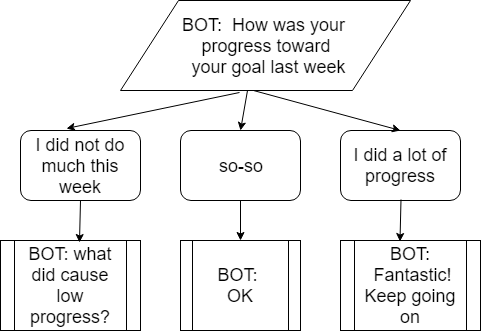Conversation diagram

It turns out, that ChatterBot has several logic adapters that allow to build conversation per different requirements.

Here is how I used logic adapter SpecificResponseAdapter for chatbot to start with initial predefined question:

``` chatbot = ChatBot("mybot",
logic_adapters=[
{
'import_path': 'chatterbot.logic.SpecificResponseAdapter',
'input_text': 'prev_day_disk',
'output_text': 'How much did you do toward your goal on previous day?'
}
.....
```

In views.py that was created in prev. post, I put input “prev_day_disk” to runbot instead of blank string. Because in the beginning of chat there is no user input and I used this to enter input_text and get desired output as specified in output_text.

```def press_my_buttons(request):
resp=""
conv=""
if request.POST:
conv=request.POST.get('conv', '')
user_input=request.POST.get('user_input', '')

userid=request.POST.get('userid', '')
if (userid == ""):
userid=uuid.uuid4()

resp=runbot(user_input, request, userid)

conv=conv + "" + str(user_input) + "\n" + "BOT:"+ str(resp) + "\n"
else:
resp=runbot("prev_day_disk", request, "")
conv =  "BOT:"+ str(resp) + "\n";

return render(request, 'my_template.html', {'conv': conv })
```

SpecificResponseAdapter can be used also in other places of conversation (not just in the beginning). For example we could use criteria if there is no input from user during 15 secs and user is not typing anything (not sure yet how easily it is to check if user typing or not) then switch conversation to new topic by making chatbot app send new question.

## How to Add Intelligence to Chatbot App

After the user replied to response how was his/her week, I want chatbot to be able to recognize the response as belonging to one of the 3 groups: bad, so-so, good. This is a machine learning classification problem. However here we will not create text classification algorithm, instead we will use built in functionality.

We will use another logic adapter, called BestMatch.
With this adapter we need specify statement_comparison_function and response_selection_method :

```chatbot = ChatBot("mybot",
logic_adapters=[
{
'import_path': 'chatterbot.logic.SpecificResponseAdapter',
'input_text': 'How much did you do toward your goal on previous day?',
'output_text': 'How much did you do toward your goal on previous day?'
},
{
"import_path": "chatterbot.logic.BestMatch",
"statement_comparison_function": "chatterbot.comparisons.levenshtein_distance",
"response_selection_method": "chatterbot.response_selection.get_first_response"
}
```

Best Match Adapter – is a logic adapter that returns a response based on known responses to the closest matches to the input statement. 

The best match adapter uses a function to compare the input statement to known statements. Once it finds the closest match to the input statement, it uses another function to select one of the known responses to that statement.

To use this adapter for the above example I need at minimum create 1 samples per each group. In the below example I used following for testing of chatbot on 2 groups (skipped so-so).

``` if (train_bot == True):
chatbot.train([
"I did not do much this week",
"Did you run into the problems with programs or just did not have time?"
])

chatbot.train([
"I did a lot of progress",
"Fantastic! Keep going on"
])
```

After the training, if the user enters something close to “I did not do much this week” the chatbot will respond with “Did you run into the problems with programs or just did not have time?”, and if user enters something like “Did a lot of progress” the bot response will be “Fantastic! Keep going on” even if the input is slightly different from training.

So we looked how to build a chatbot with logic that makes chatbot able to ask questions as needed or classify user input in several buckets and respond to user input accordingly. The code for this post is provided at the link listed below

## How to Create a Chatbot with ChatBot Open Source and Deploy It on the Web

Chatbots have become very popular due to progress in AI, ML and NLP. They are now used on many websites. With increased popularity of chatbots there are many different frameworks to create chatbot. We will explore one of such framework in this post. We will review how to create a chatbot and deploy online based on open source for ChatterBot. Our platform will be Django on pythonanywhere.

This chatbot tutorial introduces the basic concepts and terms needed to understand and deploy chatbot ChatterBot and provides a simple usage example.

## What is ChatterBot?

ChatterBot is a Python library that makes it easy to generate automated responses to a user’s input. To produce different types of responses ChatterBot uses a selection of machine learning algorithms such as search and /or classification algorithms. This makes it easy for developers to create chat bots and automate conversations with users. 

To install this chatbot open source you need just run:
pip install chatterbot

## ChatterBot Features

The functionality of chatterBot is provided through adapters – a pluggable class that allows a ChatBot instance to execute some kind of functionality.

ChatBot has the following groups of adapters
input adapters
output adapters
storage adapters
logic adapters

Inside of each group there several adapters that support different functionalities. For example within logic adapters we can use Best Match Adapter, Time Logic Adapter and few other.

Here is the example how do you run chatbot. Below is the output of running the code:

```Result of program run:
USER: How are you doing?
BOT:I am fine
USER: Hello
BOT:Hi there!
USER: Good morning!
BOT:How are today!
USER: Do you like machine learning?
BOT:Yes, I like machine learning
USER: How do I make a neural network?
BOTI am sorry, but I do not understand.
USER: Let us talk about current activities
BOT:What are working now?
USER: I am just browsing Internet for news
BOT:What a waste of time! Dont you have any other things to do?
USER: I am working on python script to make new chatbot
BOT:This is great. Keep working on this
USER: Bye
BOT:Bye
```

During the testing I confirmed that it correctly responds to new (but similar) inputs even if we did not train exactly.

Once you trained the bot, the result of training is staying there even after turning off/on PC. So if you run program multiple times, you need run training just first time. You still can run training later, for example if you want retrain or update python chatbot code.

## Deployment

This section describes how to deploy ChatterBot on pythonanywhere web hosting site with Django programming web framework.

PythonAnywhere is an online integrated development environment (IDE) and web hosting service based on the Python programming language. It has free account that allows to deploy our Chatbot. Django is needed to connect web front interface with our python code for ChatterBot in the backend. Other web frameworks like Flask can be used on pythonanywhere (instead of Django).

Below is the diagram that is showing setup that will be described below.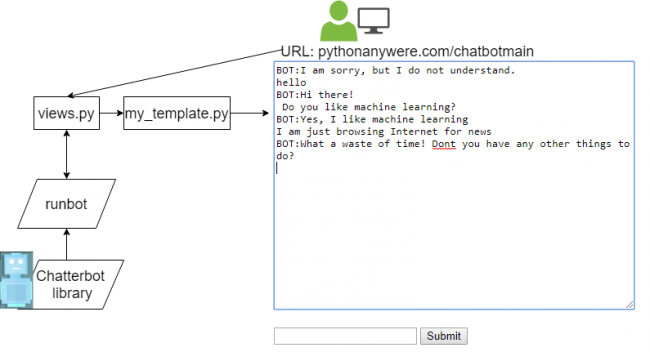Chatbot online diagram

Here is how ChatterBot can be deployed on pythonanywhere with Django:

• Go to pythonanywhere and select plan signup
• Select Create new web framework
• Select Django
• Select python version (3.6 was used in this example)
• Select project name directory
• It will create the following:
/home/user/project_name
manage.py
my_template.html
views.py
——————-project_name
init_.py
settings.py
urls.py
wsgi.py

• create folder cbot under user folder
• /home/user/cbot

• inside of this folder create
• __init__.py (this is just empty file)
chatbotpy.py

Inside chatbotpy wrap everything into function runbot like below. In this function we are initiating chatbot object, taking user input, if needed we train chatbot and then asking for chatbot response. The response provided by chatbot is the output of this function. The input of this function is the user input that we are getting through web.

```def runbot(inp, train_bot=False):
from chatterbot import ChatBot

chatbot = ChatBot("mychatbot",
logic_adapters=[
{
"import_path": "chatterbot.logic.BestMatch",
"statement_comparison_function": "chatterbot.comparisons.levenshtein_distance",
"response_selection_method": "chatterbot.response_selection.get_first_response"
},
{
'import_path': 'chatterbot.logic.LowConfidenceAdapter',
'threshold': 0.65,
'default_response': 'I am sorry, but I do not understand.'
}
],
trainer='chatterbot.trainers.ListTrainer')

if (train_bot == True):
print "Training"
"""
Insert here training code from the python code for ChatterBot example
"""

response = chatbot.get_response(inp)
return (response)
```

Now update views.py like below code box. Here we are taking user input from web, feeding this input to runbot function and sending output of runbot function (which is chatbot reponse) to web template.

```
from django.shortcuts import render
from cbot.chatbotpy import runbot

def press_my_buttons(request):
resp=""
conv=""
if request.POST:
conv=request.POST.get('conv', '')
user_input=request.POST.get('user_input', '')

resp=runbot(user_input)

conv=conv + "" + str(user_input) + "\n" + "BOT:"+ str(resp) + "\n"
else:
resp=runbot("")
conv =  "BOT:"+ str(resp) + "\n";

return render(request, 'my_template.html', {'conv': conv})
```

Now update my_template.html like below. Here we just show new response together with previous conversation information.

```<html>
<form method="post">
{% csrf_token %}

<textarea rows=20 cols=60>{{conv}}</textarea>

<br><br>
<input type="textbox" name="user_input" value=""/>

<button type="submit">Submit</button>

<input type="hidden" name =conv  value="{{conv}}" />
{{resp}}
</form>
</html>
```

Now update some configuration.
Update or confirm manage.py to include the line with settings.

```if __name__ == '__main__':
os.environ.setdefault('DJANGO_SETTINGS_MODULE', 'cbotdjango.settings')
```

Update or confirm urls.py to have path like below

```import sys
path = "/home/user/cbotdjango"
if path not in sys.path:
sys.path.append(path)
```

Now you are ready do testing chatbot online and should see the screen similar in the setup diagram on right side.

## Conclusion

We saw how to train ChatterBot and some functionality of it. We investigated how to install ChatterBot on pythonanywhere with Django web framework. Hope this will make easy to deploy chatbot in case you are going this route. If you have any tips or anything else to add, please leave a comment below.

References
1. ChatterBot
2. Python chatbot code example
3. Python Anywhere

## Text Clustering with doc2vec Word Embedding Machine Learning ModelIn this post we will look at doc2vec word embedding model, how to build it or use pretrained embedding file. For practical example we will explore how to do text clustering with doc2vec model.

## Doc2vec

Doc2vec is an unsupervised computer algorithm to generate vectors for sentence/paragraphs/documents. The algorithm is an adaptation of word2vec which can generate vectors for words. Below you can see frameworks for learning word vector word2vec (left side) and paragraph vector doc2vec (right side). For learning doc2vec, the paragraph vector was added to represent the missing information from the current context and to act as a memory of the topic of the paragraph.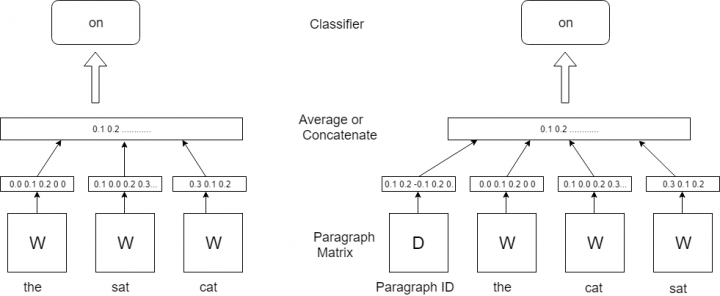Word Embeddings Machine Learning Frameworks: word2vec and doc2vec

If you need information about word2vec here are some posts:
word2vec –
Vector Representation of Text – Word Embeddings with word2vec
word2vec application –
Text Analytics Techniques with Embeddings
Using Pretrained Word Embeddinigs in Machine Learning
K Means Clustering Example with Word2Vec in Data Mining or Machine Learning

The vectors generated by doc2vec can be used for tasks like finding similarity between sentences / paragraphs / documents.  With doc2vec you can get vector for sentence or paragraph out of model without additional computations as you would do it in word2vec, for example here we used function to go from word level to sentence level:
Text Clustering with Word Embedding in Machine Learning

word2vec was very successful and it created idea to convert many other specific texts to vector. It can called “anything to vector”. So there are many different word embedding models that like doc2vec can convert more than one word to numeric vector.  Here are few examples:

tweet2vec Tweet2Vec: Character-Based Distributed Representations for Social Media
lda2vec Mixing Dirichlet Topic Models and Word Embeddings to Make lda2vec. Here is proposed model that learns dense word vectors jointly with Dirichlet-distributed latent document-level mixtures of topic vectors.
Topic2Vec Learning Distributed Representations of Topics
Med2vec Multi-layer Representation Learning for Medical Concepts
The list can go on. In the next section we will look how to load doc2vec and use for text clustering.

## Building doc2vec Model

Here is the example for converting word paragraph to vector using own built doc2vec model. The example is taken from .

The script consists of the following main steps:

• build model using own text
• save model to file
• load model from this file
• infer vector representation
```
from gensim.test.utils import common_texts
from gensim.models.doc2vec import Doc2Vec, TaggedDocument

print (common_texts)

"""
output:
[['human', 'interface', 'computer'], ['survey', 'user', 'computer', 'system', 'response', 'time'], ['eps', 'user', 'interface', 'system'], ['system', 'human', 'system', 'eps'], ['user', 'response', 'time'], ['trees'], ['graph', 'trees'], ['graph', 'minors', 'trees'], ['graph', 'minors', 'survey']]
"""

documents = [TaggedDocument(doc, [i]) for i, doc in enumerate(common_texts)]

print (documents)
"""
output
[TaggedDocument(words=['human', 'interface', 'computer'], tags=), TaggedDocument(words=['survey', 'user', 'computer', 'system', 'response', 'time'], tags=), TaggedDocument(words=['eps', 'user', 'interface', 'system'], tags=), TaggedDocument(words=['system', 'human', 'system', 'eps'], tags=), TaggedDocument(words=['user', 'response', 'time'], tags=), TaggedDocument(words=['trees'], tags=), TaggedDocument(words=['graph', 'trees'], tags=), TaggedDocument(words=['graph', 'minors', 'trees'], tags=), TaggedDocument(words=['graph', 'minors', 'survey'], tags=)]

"""

model = Doc2Vec(documents, size=5, window=2, min_count=1, workers=4)
#Persist a model to disk:

from gensim.test.utils import get_tmpfile
fname = get_tmpfile("my_doc2vec_model")

print (fname)
#output: C:\Users\userABC\AppData\Local\Temp\my_doc2vec_model

#load model from saved file
model.save(fname)
model = Doc2Vec.load(fname)
# you can continue training with the loaded model!
#If you’re finished training a model (=no more updates, only querying, reduce memory usage), you can do:

model.delete_temporary_training_data(keep_doctags_vectors=True, keep_inference=True)

#Infer vector for a new document:
#Here our text paragraph just 2 words
vector = model.infer_vector(["system", "response"])
print (vector)

"""
output

[-0.08390492  0.01629403 -0.08274432  0.06739668 -0.07021132]

"""
```

## Using Pretrained doc2vec Model

We can skip building embedding file step and use already built file. Here is an example how to do coding with pretrained word embedding file for representing test docs as vectors. The script is based on .

The below script is using pretrained on Wikipedia data doc2vec model from this location

Here is the link where you can find links to different pre-trained doc2vec and word2vec models and additional information.

You need to download zip file, unzip , put 3 files at some folder and provide path in the script. In this example it is “doc2vec/doc2vec.bin”

The main steps of the below script consist of just load doc2vec model and infer vectors.

```
import gensim.models as g
import codecs

model="doc2vec/doc2vec.bin"
test_docs="data/test_docs.txt"
output_file="data/test_vectors.txt"

#inference hyper-parameters
start_alpha=0.01
infer_epoch=1000

#load model
m = g.Doc2Vec.load(model)
test_docs = [ x.strip().split() for x in codecs.open(test_docs, "r", "utf-8").readlines() ]

#infer test vectors
output = open(output_file, "w")
for d in test_docs:
output.write( " ".join([str(x) for x in m.infer_vector(d, alpha=start_alpha, steps=infer_epoch)]) + "\n" )
output.flush()
output.close()

"""
output file
0.03772797 0.07995503 -0.1598981 0.04817521 0.033129826 -0.06923918 0.12705861 -0.06330753 .........
"""
```

So we got output file with vectors (one per each paragraph). That means we successfully converted our text to vectors. Now we can use it for different machine learning algorithms such as text classification, text clustering and many other. Next section will show example for Birch clustering algorithm with word embeddings.

## Using Pretrained doc2vec Model for Text Clustering (Birch Algorithm)

In this example we use Birch clustering algorithm for clustering text data file from 
Birch is unsupervised algorithm that is used for hierarchical clustering. An advantage of this algorithm is its ability to incrementally and dynamically cluster incoming data 

We use the following steps here:

• Load doc2vec model
• Load text docs that will be clustered
• Convert docs to vectors (infer_vector)
• Do clustering
```from sklearn import metrics

import gensim.models as g
import codecs

model="doc2vec/doc2vec.bin"
test_docs="data/test_docs.txt"

#inference hyper-parameters
start_alpha=0.01
infer_epoch=1000

#load model
m = g.Doc2Vec.load(model)
test_docs = [ x.strip().split() for x in codecs.open(test_docs, "r", "utf-8").readlines() ]

print (test_docs)
"""
[['the', 'cardigan', 'welsh', 'corgi'........
"""

X=[]
for d in test_docs:

X.append( m.infer_vector(d, alpha=start_alpha, steps=infer_epoch) )

k=3

from sklearn.cluster import Birch

brc = Birch(branching_factor=50, n_clusters=k, threshold=0.1, compute_labels=True)
brc.fit(X)

clusters = brc.predict(X)

labels = brc.labels_

print ("Clusters: ")
print (clusters)

silhouette_score = metrics.silhouette_score(X, labels, metric='euclidean')

print ("Silhouette_score: ")
print (silhouette_score)

"""
Clusters:
[1 0 0 1 1 2 1 0 1 1]
Silhouette_score:
0.17644188
"""

```

If you want to get some test with text clustering and word embeddings here is the online demo Currently it is using word2vec and glove models and k means clustering algorithm. Select ‘Text Clustering’ option and scroll down to input data.

## Conclusion

We looked what is doc2vec is, we investigated 2 ways to load this model: we can create embedding model file from our text or use pretrained embedding file. We applied doc2vec to do Birch algorithm for text clustering. In case we need to work with paragraph / sentences / docs, doc2vec can simplify word embedding for converting text to vectors.

## Text Clustering with Word Embedding in Machine Learning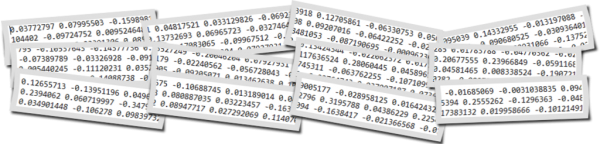Text clustering is widely used in many applications such as recommender systems, sentiment analysis, topic selection, user segmentation. Word embeddings (for example word2vec) allow to exploit ordering
of the words and semantics information from the text corpus. In this blog you can find several posts dedicated different word embedding models:

In contrast to last post from the above list, in this post we will discover how to do text clustering with word embeddings at sentence (phrase) level. The sentence could be a few words, phrase or paragraph like tweet. For examples we have 1000 of tweets and want to group in several clusters. So each cluster would contain one or more tweets.

## Data

Our data will be the set of sentences (phrases) containing 2 topics as below:
Note: I highlighted in bold 3 sentences on weather topic, all other sentences have totally different topic.
sentences = [[‘this’, ‘is’, ‘the’, ‘one’,’good’, ‘machine’, ‘learning’, ‘book’],
[‘this’, ‘is’, ‘another’, ‘book’],
[‘one’, ‘more’, ‘book’],
[‘weather’, ‘rain’, ‘snow’],
[‘yesterday’, ‘weather’, ‘snow’],
[‘forecast’, ‘tomorrow’, ‘rain’, ‘snow’],

[‘this’, ‘is’, ‘the’, ‘new’, ‘post’],
[‘this’, ‘is’, ‘about’, ‘more’, ‘machine’, ‘learning’, ‘post’],
[‘and’, ‘this’, ‘is’, ‘the’, ‘one’, ‘last’, ‘post’, ‘book’]]

## Word Embedding Method

For embeddings we will use gensim word2vec model. There is also doc2vec model – but we will use it at next post.
With the need to do text clustering at sentence level there will be one extra step for moving from word level to sentence level. For each sentence from the set of sentences, word embedding of each word is summed and in the end divided by number of words in the sentence. So we are getting average of all word embeddings for each sentence and use them as we would use embeddings at word level – feeding to machine learning clustering algorithm such k-means.

Here is the example of the function that doing this:

```def sent_vectorizer(sent, model):
sent_vec =[]
numw = 0
for w in sent:
try:
if numw == 0:
sent_vec = model[w]
else:
sent_vec = np.add(sent_vec, model[w])
numw+=1
except:
pass

return np.asarray(sent_vec) / numw
```

Now we will use text clustering Kmeans algorithm with word2vec model for embeddings. For kmeans algorithm we will use 2 separate implementations with different libraries NLTK for KMeansClusterer and sklearn for cluster. This was described in previous posts (see the list above).

The code for this article can be found in the end of this post. We use 2 for number of clusters in both k means text clustering algorithms.
Additionally we will plot data using tSNE.

## Output

Below are results

[1, 1, 1, 0, 0, 0, 1, 1, 1]

Cluster id and sentence:
1:[‘this’, ‘is’, ‘the’, ‘one’, ‘good’, ‘machine’, ‘learning’, ‘book’]
1:[‘this’, ‘is’, ‘another’, ‘book’]
1:[‘one’, ‘more’, ‘book’]
0:[‘weather’, ‘rain’, ‘snow’]
0:[‘yesterday’, ‘weather’, ‘snow’]
0:[‘forecast’, ‘tomorrow’, ‘rain’, ‘snow’]

1:[‘this’, ‘is’, ‘the’, ‘new’, ‘post’]
1:[‘this’, ‘is’, ‘about’, ‘more’, ‘machine’, ‘learning’, ‘post’]
1:[‘and’, ‘this’, ‘is’, ‘the’, ‘one’, ‘last’, ‘post’, ‘book’]

Score (Opposite of the value of X on the K-means objective which is Sum of distances of samples to their closest cluster center):
-0.0008175040203510163
Silhouette_score:
0.3498247

Cluster id and sentence:
1 [‘this’, ‘is’, ‘the’, ‘one’, ‘good’, ‘machine’, ‘learning’, ‘book’]
1 [‘this’, ‘is’, ‘another’, ‘book’]
1 [‘one’, ‘more’, ‘book’]
0 [‘weather’, ‘rain’, ‘snow’]
0 [‘yesterday’, ‘weather’, ‘snow’]
0 [‘forecast’, ‘tomorrow’, ‘rain’, ‘snow’]

1 [‘this’, ‘is’, ‘the’, ‘new’, ‘post’]
1 [‘this’, ‘is’, ‘about’, ‘more’, ‘machine’, ‘learning’, ‘post’]
1 [‘and’, ‘this’, ‘is’, ‘the’, ‘one’, ‘last’, ‘post’, ‘book’]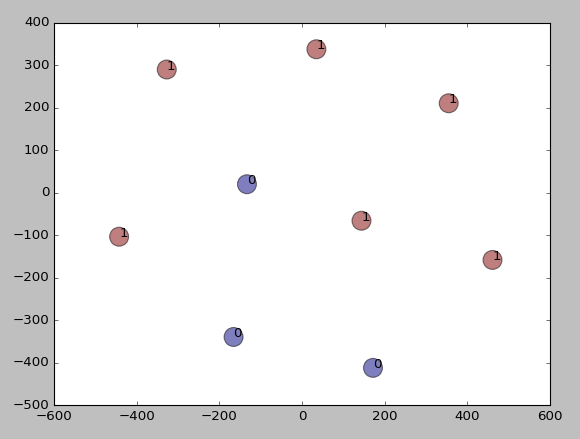Results of text clustering

We see that the data were clustered according to our expectation – different sentences by topic appeared to different clusters. Thus we learned how to do clustering algorithms in data mining or machine learning with word embeddings at sentence level. Here we used kmeans clustering and word2vec embedding model. We created additional function to go from word embeddings to sentence embeddings level. In the next post we will use doc2vec and will not need this function.

Below is full source code python script.

```from gensim.models import Word2Vec

from nltk.cluster import KMeansClusterer
import nltk
import numpy as np

from sklearn import cluster
from sklearn import metrics

# training data

sentences = [['this', 'is', 'the', 'one','good', 'machine', 'learning', 'book'],
['this', 'is',  'another', 'book'],
['one', 'more', 'book'],
['weather', 'rain', 'snow'],
['yesterday', 'weather', 'snow'],
['forecast', 'tomorrow', 'rain', 'snow'],
['this', 'is', 'the', 'new', 'post'],
['this', 'is', 'about', 'more', 'machine', 'learning', 'post'],
['and', 'this', 'is', 'the', 'one', 'last', 'post', 'book']]

model = Word2Vec(sentences, min_count=1)

def sent_vectorizer(sent, model):
sent_vec =[]
numw = 0
for w in sent:
try:
if numw == 0:
sent_vec = model[w]
else:
sent_vec = np.add(sent_vec, model[w])
numw+=1
except:
pass

return np.asarray(sent_vec) / numw

X=[]
for sentence in sentences:
X.append(sent_vectorizer(sentence, model))

print ("========================")
print (X)

# note with some version you would need use this (without wv)
#  model[model.vocab]
print (model[model.wv.vocab])

print (model.similarity('post', 'book'))
print (model.most_similar(positive=['machine'], negative=[], topn=2))

NUM_CLUSTERS=2
kclusterer = KMeansClusterer(NUM_CLUSTERS, distance=nltk.cluster.util.cosine_distance, repeats=25)
assigned_clusters = kclusterer.cluster(X, assign_clusters=True)
print (assigned_clusters)

for index, sentence in enumerate(sentences):
print (str(assigned_clusters[index]) + ":" + str(sentence))

kmeans = cluster.KMeans(n_clusters=NUM_CLUSTERS)
kmeans.fit(X)

labels = kmeans.labels_
centroids = kmeans.cluster_centers_

print ("Cluster id labels for inputted data")
print (labels)
print ("Centroids data")
print (centroids)

print ("Score (Opposite of the value of X on the K-means objective which is Sum of distances of samples to their closest cluster center):")
print (kmeans.score(X))

silhouette_score = metrics.silhouette_score(X, labels, metric='euclidean')

print ("Silhouette_score: ")
print (silhouette_score)

import matplotlib.pyplot as plt

from sklearn.manifold import TSNE

model = TSNE(n_components=2, random_state=0)
np.set_printoptions(suppress=True)

Y=model.fit_transform(X)

plt.scatter(Y[:, 0], Y[:, 1], c=assigned_clusters, s=290,alpha=.5)

for j in range(len(sentences)):
plt.annotate(assigned_clusters[j],xy=(Y[j], Y[j]),xytext=(0,0),textcoords='offset points')
print ("%s %s" % (assigned_clusters[j],  sentences[j]))

plt.show()
```

## Topic Modeling Python and Textacy Example

Topic modeling is automatic discovering the abstract “topics” that occur in a collection of documents. It can be used for providing more informative view of search results, quick overview for set of documents or some other services.

## Textacy

In this post we will look at topic modeling with textacy. Textacy is a Python library for performing a variety of natural language processing (NLP) tasks, built on the high-performance spacy library.
It can flexibly tokenize and vectorize documents and corpora, then train, interpret, and visualize topic models using LSA, LDA, or NMF methods. 
Textacy is less known than other python libraries such as NLTK, SpaCY, TextBlob  But it looks very promising as it’s built on the top of spaCY.

In this post we will use textacy for the following task. We have group of documents and we want extract topics out of this set of documents. We will use 20 Newsgroups dataset as the source of documents.

## Code Structure

Our code consist of the following steps:
Get data. We will use only 2 groups (alt.atheism’, ‘soc.religion.christian’).
Tokenize and remove some not needed characters or stopwords.
Vectorize.
Extract Topics. Here we do actual topic modeling. We use Non-negative Matrix Factorization method. (NMF)
Output graph of terms – topic matrix.

## Output

Below is the final output plot.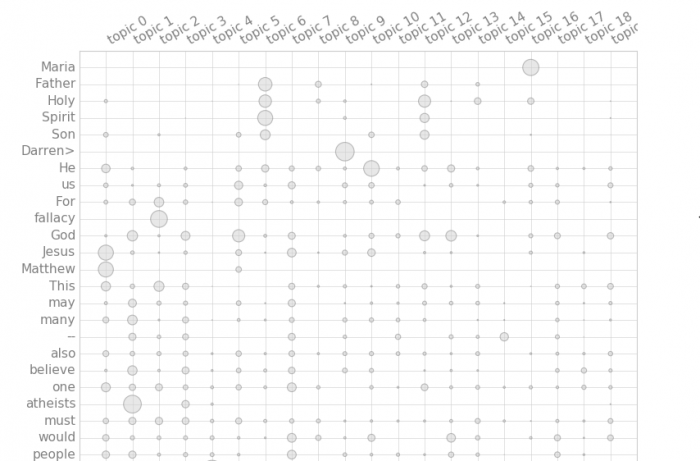Topic modeling with textacy

Looking at output graph we can see term distribution over the topics. We identified more than 2 topics. For example topic 2 is associated with atheism, while topic 1 is associated with God, religion.

While better data preparation is needed to remove few more non meaningful words, the example still showing that to do topic modeling with textacy is much easy than with some other modes (for example gensim). This is because it has ability to do many things that you need do after NLP versus just do NLP and allow user then add additional data views, heatmaps or diagrams.

Here are few links with topic modeling using LDA and gensim (not using textacy). The posts demonstrate that it is required more coding comparing with textacy.
Topic Extraction from Blog Posts with LSI , LDA and Python
Data Visualization – Visualizing an LDA Model using Python

## Source Code

Below is python full source code.

```categories = ['alt.atheism', 'soc.religion.christian']

#Loading the data set - training data.
from sklearn.datasets import fetch_20newsgroups

newsgroups_train = fetch_20newsgroups(subset='train', shuffle=True, categories=categories, remove=('headers', 'footers', 'quotes'))

# You can check the target names (categories) and some data files by following commands.
print (newsgroups_train.target_names) #prints all the categories
print("\n".join(newsgroups_train.data.split("\n")[:3])) #prints first line of the first data file
print (newsgroups_train.target_names)
print (len(newsgroups_train.data))

texts = []

labels=newsgroups_train.target
texts = newsgroups_train.data

from nltk.corpus import stopwords

import textacy
from textacy.vsm import Vectorizer

terms_list=[[tok  for tok in doc.split() if tok not in stopwords.words('english') ] for doc in texts]

count=0
for doc in terms_list:
for word in doc:
print (word)
if word == "|>" or word == "|>" or word == "_" or word == "-" or word == "#":
terms_list[count].remove (word)
if word == "=":
terms_list[count].remove (word)
if word == ":":
terms_list[count].remove (word)
if word == "_/":
terms_list[count].remove (word)
if word == "I" or word == "A":
terms_list[count].remove (word)
if word == "The" or word == "But" or word=="If" or word=="It":
terms_list[count].remove (word)
count=count+1

print ("=====================terms_list===============================")
print (terms_list)

vectorizer = Vectorizer(tf_type='linear', apply_idf=True, idf_type='smooth')
doc_term_matrix = vectorizer.fit_transform(terms_list)

print ("========================doc_term_matrix)=======================")
print (doc_term_matrix)

#initialize and train a topic model:
model = textacy.tm.TopicModel('nmf', n_topics=20)
model.fit(doc_term_matrix)

print ("======================model=================")
print (model)

doc_topic_matrix = model.transform(doc_term_matrix)
for topic_idx, top_terms in model.top_topic_terms(vectorizer.id_to_term, topics=[0,1]):
print('topic', topic_idx, ':', '   '.join(top_terms))

for i, val in enumerate(model.topic_weights(doc_topic_matrix)):
print(i, val)

print   ("doc_term_matrix")
print   (doc_term_matrix)
print ("vectorizer.id_to_term")
print (vectorizer.id_to_term)

model.termite_plot(doc_term_matrix, vectorizer.id_to_term, topics=-1,  n_terms=25, sort_terms_by='seriation')
model.save('nmf-10topics.pkl')

```

## Text Mining Techniques for Search Results Clustering

Text search box can be found almost in every web based application that has text data. We use search feature when we are looking for customer data, jobs descriptions, book reviews or some other information. Simple keyword matching can be enough in some small tasks. However when we have many results something better than keyword match would be very helpful. Instead of going through a lot of results we would get results grouped by topic with a nice summary of topics. It would allow to see information at first sight.

In this post we will look in some machine learning algorithms, applications and frameworks that can analyze output of search function and provide useful additional information for search results.

## Machine Learning Clustering for Search Results

Search results clustering problem is defined as an automatic, on-line grouping of similar documents in a search results list returned from a search engine.  Carrot2 is the tool that was built to solve this problem.
Carrot2 is Open Source Framework for building Search Results Clustering Engine. This tool can do search, cluster and visualize clusters. Which is very cool. I was not able to find similar like this tool in the range of open source projects. If you are aware of such tool, please suggest in the comment box.

Below are screenshots of clustering search results from Carrot2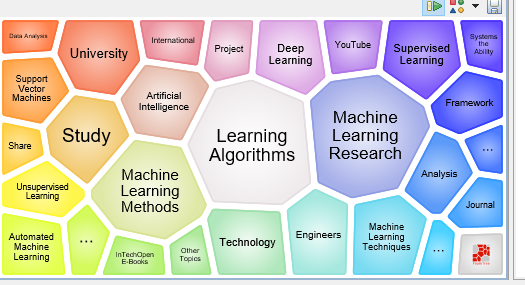Clustering search results with Carrot2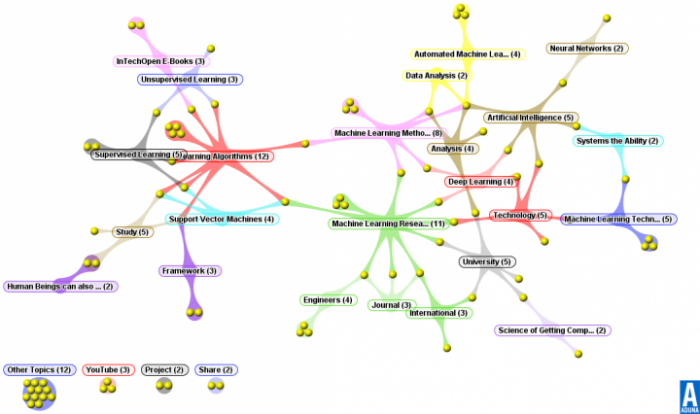Aduna cluster map visualization clusters with Carrot2

The following algorithms are behind Carrot2 tool:
Lingo algorithm constructs a “term-document matrix” where each snippet gets a column, each word a row and the values are the frequency of that word in that snippet. It then applies a matrix factorization called singular value decomposition or SVD. 

Suffix Tree Clustering (STC) uses the generalised suffix tree data structure, to efficiently build a list of the most frequently used phrases in the snippets from the search results. 

## Topic modelling

Topic modelling is another approach that is used to identify which topic is discussed in documents or text snippets provided by search function. There are several methods like LSA, pLSA, LDA 

Comprehensive overview of Topic Modeling and its associated techniques is described in 

Topic modeling can be represented via below diagram. Our goal is identify topics given documents with the words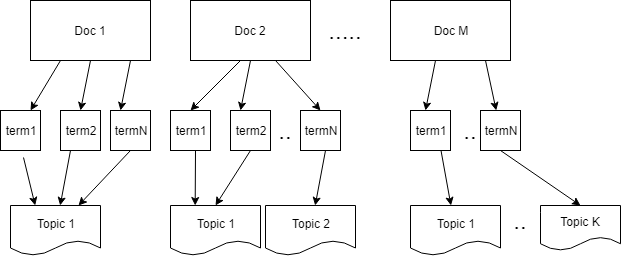Topic modeling diagram

Below is plate notation of LDA model.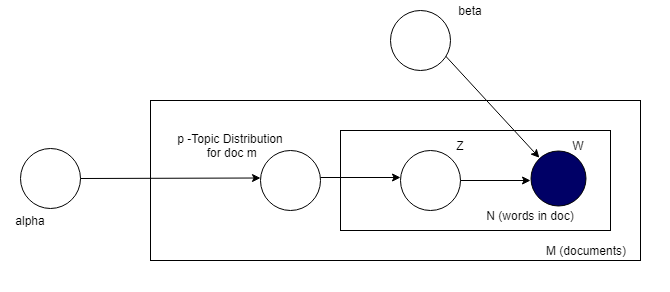Plate notation of LDA model

Plate notation representing the LDA model. 
αlpha is the parameter of the Dirichlet prior on the per-document topic distributions,
βeta is the parameter of the Dirichlet prior on the per-topic word distribution,
p is the topic distribution for document m,
Z is the topic for the n-th word in document m, and
W is the specific word.

We can use different NLP libraries (NLTK, spaCY, gensim, textacy) for topic modeling.
Here is the example of topic modeling with textacy python library:
Topic Modeling Python and Textacy Example

Here are examples of topic modeling with gensim library:
Topic Extraction from Blog Posts with LSI , LDA and Python
Data Visualization – Visualizing an LDA Model using Python

## Using Word Embeddings

Word embeddings like gensim, word2vec, glove showed very good results in NLP and are widely used now. This is also used for search results clustering. The first step would be create model for example gensim. In the next step text data are converted to vector representation. Words embedding improve preformance by leveraging information on how words are semantically correlated to each other 

## Neural Topic Model (NTM) and Other Approaches

Below are some other approaches that can be used for topic modeling for search results organizing.
Neural topic modeling – combines a neural network with a latent topic model. 
Topic modeling with Deep Belief Nets is described in . The concept of the method is to load bag-of-words (BOW) and produce a strong latent representation that will then be used for a content based recommender system. The authors report that model outperform LDA, Replicated Softmax, and DocNADE models on document retrieval and document classification tasks.

Thus we looked at different techniques for search results clustering. In the future posts we will implement some of them. What machine learning methods do you use for presenting search results? I would love to hear.